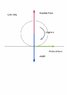# Car movement physics

htincho
I want to model a car movement.

The equations are:

$$\sum$$F = ma

$$\sum$$T = m $$\alpha$$

The forces are, weight of the car, normal force and f the friccional force, but also the engine provides a constant rotational acceleration in my model.

I understand that the frictional force is the one that moves the car, so:

f = ma

But also the movement of the engine generates Torque, and I can't realize how to connect this with the torque of the friccional force. Because if the car engine stop the frictional force stop the wheel but when the car engine is connected to the wheel they must oppose.

It's correct to say something like:
Torque (of car engine) - Tf (of frictional force) = I$$\alpha$$

¿Does the frictional forces opposes to the rotation of the wheel car in the rotational movement??

I can't complete the movement description.

htincho
I can't edit the post, the formulas are "wrong" because I never use latex.

I want to solve the problem thinking of the tire as a solid ¿body? (I don't know how translate it) using these equations.

$$\sum F = ma$$

$$\sum T = I \alpha$$

I know the Torque of the car and I want to know how this is translated into equations, I've think something like:

$$\f (frictionalForce) = ma$$

$$\sum T(engine) - T(frictionalForce) = I \alpha$$

But I'm not sure if it's right to express the Torque of the car in that way.

#### Attachments

•carTire.jpg
7.3 KB · Views: 382
Homework Helper
Welcome to PF!

I know the Torque of the car and I want to know how this is translated into equations, I've think something like:

$$\f (frictionalForce) = ma$$

$$\sum T(engine) - T(frictionalForce) = I \alpha$$

Hi htincho! Welcome to PF!(copy-and-paste the symbols below for future use …)

I suspect you're misunderstanding how friction affects wheels.

Friction helps wheels to move (wheels don't work on ice, do they?).

The only friction resisting movement of the car is air resistance plus the friction of the internal parts of the car.htincho
Thank you for the welcome and thank you for answering, but I can't understand you completely what you are saying.

On ice the car doesn't move but the tire must spin if you're accelerating, so I don't know how to connect the engine torque that's it is providing to the wheel so that it can spin without moving on ice. That's why I added a Torque for engine. The frictional force in the Newton third law is the one that gives the acceleration but it also generates torque. At least that's what I'm thinking because that frictional force is applied over the surface and I'm taking moment over the center of the tire. The torque in the equation was negative because of the coordinate system that I doesn't draw.

On ice the rotational coefficient tends to 0, so that no force is applying. But in other cases there must be some rotational coefficient.

$$f = rollingFriction$$

$$Tf = torqueOfRollingFriction$$

$$Te = torqueOfEngine$$

$$f = ma$$

$$Tf - Te = I\alpha$$

$$Tf = \mu_{r} N$$

I know that there is friction from air and that's really important but that depends on the speed of the car, so if it's moving very slow the air friction could be avoided and the internal part of the car also have but I just want to understand this first part.

#### Attachments

Homework Helper
Hi htincho!Imagine that the car is on ordinary ground, with the engine in forward gear, but that the wheel is slipping (so there's no acceleration).

Then the frictional force on the car will be µW (the weight times the coefficient of friction between the tyre and the ground).

And it will be forward!

Now slow the engine, so that the wheel isn't slipping … the friction force will still be forward, won't it?

The friction force is what is pushing the car!

The friction force is the lesser of µW and Tr, where T is torque and r is radius.

In other words: if the wheel is not slipping, the force which is pushing the car is the friction force, which always equals Tr.